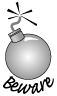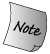### 1.2. 初窥输入/输出

C++ does not directly define any statements to do input or output (IO). Instead, IO is provided by the standard library. The IO library provides an extensive set offacilities. However, for many purposes, including the examples in this book, one needs to know only a few basic concepts and operations.

C++ 并没有直接定义进行输入或输出（IO）的任何语句，这种功能是由标准库提供的。IO 库提供了大量的设施。然而，对许多应用，包括本书的例子而言，编程者只需要了解一些基本概念和操作。

Most of the examples in this book use the iostream library, which handles formatted input and output. Fundamental to the iostream library are two types named istream and ostream, which represent input and output streams, respectively. A stream is a sequence of characters intended to be read from or written to an IO device of some kind. The term "stream" is intended to suggest that the characters are generated, or consumed, sequentially over time.

#### 1.2.1. 标准输入与输出对象

The library defines four IO objects. To handle input, we use an object of type istream named cin (pronounced "see-in"). This object is also referred to as the standard input. For output, we use an ostream object named cout (pronounced "see-out"). It is often referred to as the standard output. The library also defines two other ostream objects, named cerr and clog (pronounced "see-err" and "see-log," respectively). The cerr object, referred to as the standard error, is typically used to generate warning and error messages to users of our programs. The clog object is used for general information about the execution of the program.

Ordinarily, the system associates each of these objects with the window in which the program is executed. So, when we read from cin, data is read from the window in which the program is executing, and when we write to cout, cerr, or clog, the output is written to the same window. Most operating systems give us a way of redirecting the input or output streams when we run a program. Using redirection we can associate these streams with files of our choosing.

#### 1.2.2. 一个使用IO库的程序

So far, we have seen how to compile and execute a simple program, although that program did no work. In our overall problem, we'll have several records that refer to the same ISBN. We'll need to consolidate those records into a single total, implying that we'll need to know how to add the quantities of books sold.

To see how to solve part of that problem, let's start by looking at how we might add two numbers. Using the IO library, we can extend our main program to ask the user to give us two numbers and then print their sum:

```    #include <iostream>
int main()
{
std::cout << "Enter two numbers:" << std::endl;
int v1, v2;
std::cin >> v1 >> v2;
std::cout << "The sum of " << v1 << " and " << v2
<< " is " << v1 + v2 << std::endl;
return 0;
}
```

This program starts by printing

```      Enter two numbers:
```

on the user's screen and then waits for input from the user. If the user enters

```      3 7
```

followed by a newline, then the program produces the following output:

```      The sum of 3 and 7 is 10
```

The first line of our program is a preprocessor directive:

```      #include <iostream>
```

which tells the compiler that we want to use the iostream library. The name inside angle brackets is a header. Every program that uses a library facility must include its associated header. The #include directive must be written on a single linethe name of the header and the #include must appear on the same line. In general, #include directives should appear outside any function. Typically, all the #include directives for a program appear at the beginning of the file.

##### 写入到流

The first statement in the body of main executes an expression. In C++ an expression is composed of one or more operands and (usually) an operator. The expressions in this statement use the output operator (the << operator) to print the prompt on the standard output:

main 函数体中第一条语句执行了一个表达式。C++ 中，一个表达式由一个或几个操作数和通常是一个操作符组成。该语句的表达式使用输出操作符<< 操作符），在标准输出上输出提示语：

```      std::cout << "Enter two numbers:" << std::endl;
```

This statement uses the output operator twice. Each instance of the output operator takes two operands: The left-hand operand must be an ostream object; the right-hand operand is a value to print. The operator writes its right-hand operand to the ostream that is its left-hand operand.

In C++ every expression produces a result, which typically is the value generated by applying an operator to its operands. In the case of the output operator, the result is the value of its left-hand operand. That is, the value returned by an output operation is the output stream itself.

C++ 中，每个表达式都会产生一个结果，通常是将操作符作用到其操作数所产生的值。当操作符是输出操作符时，结果是左操作数的值。也就是说，输出操作返回的值是输出流本身。

The fact that the operator returns its left-hand operand allows us to chain together output requests. The statement that prints our prompt is equivalent to

```      (std::cout << "Enter two numbers:") << std::endl;
```

Because (std::cout << "Enter two numbers:") returns its left operand, std::cout, this statement is equivalent to

```      std::cout << "Enter two numbers:";
std::cout << std::endl;
```

endl is a special value, called a manipulator, that when written to an output stream has the effect of writing a newline to the output and flushing the buffer associated with that device. By flushing the buffer, we ensure that the user will see the output written to the stream immediately.

endl 是一个特殊值，称为操纵符，将它写入输出流时，具有输出换行的效果，并刷新与设备相关联的缓冲区。通过刷新缓冲区，用户可立即看到写入到流中的输出。Programmers often insert print statements during debugging. Such statements should always flush the stream. Forgetting to do so may cause output to be left in the buffer if the program crashes, leading to incorrect inferences about where the program crashed. 程序员经常在调试过程中插入输出语句，这些语句都应该刷新输出流。忘记刷新输出流可能会造成输出停留在缓冲区中，如果程序崩溃，将会导致程序错误推断崩溃位置。
##### 使用标准库中的名字

Careful readers will note that this program uses std::cout and std::endl rather than just cout and endl. The prefix std:: indicates that the names cout and endl are defined inside the namespace named std. Namespaces allow programmers to avoid inadvertent collisions with the same names defined by a library. Because the names that the standard library defines are defined in a namespace, we can use the same names for our own purposes.

One side effect of the library's use of a namespace is that when we use a name from the library, we must say explicitly that we want to use the name from the std namespace. Writing std::cout uses the scope operator (the :: operator) to say that we want to use the name cout that is defined in the namespace std. We'll see in Section 3.1 (p. 78) a way that programs often use to avoid this verbose syntax.

##### 读入流

Having written our prompt, we next want to read what the user writes. We start by defining two variables named v1 and v2 to hold the input:

```      int v1, v2;
```

We define these variables as type int, which is the built-in type representing integral values. These variables are uninitialized, meaning that we gave them no initial value. Our first use of these variables will be to read a value into them, so the fact that they have no initial value is okay.

The next statement

```      std::cin >> v1 >> v2;
```

reads the input. The input operator (the >> operator) behaves analogously to the output operator. It takes an istream as its left-hand operand and an object as its right-hand operand. It reads from its istream operand and stores the value it read in its right-hand operand. Like the output operator, the input operator returns its left-hand operand as its result. Because the operator returns its left-hand operand, we can combine a sequence of input requests into a single statement. In other words, this input operation is equivalent to

```      std::cin >> v1;
std::cin >> v2;
```

The effect of our input operation is to read two values from the standard input, storing the first in v1 and the second in v2.

##### 完成程序

What remains is to print our result:

```     std::cout << "The sum of " << v1 << " and " << v2
<< " is " << v1 + v2 << std::endl;
```

This statement, although it is longer than the statement that printed the prompt, is conceptually no different. It prints each of its operands to the standard output. What is interesting is that the operands are not all the same kinds of values. Some operands are string literals, such as

```   "The sum of "
```

and others are various int values, such as v1, v2, and the result of evaluating the arithmetic expression:

```   v1 + v2
```

The iostream library defines versions of the input and output operators that accept all of the built-in types.When writing a C++ program, in most places that a space appears we could instead use a newline. One exception to this rule is that spaces inside a string literal cannot be replaced by a newline. Another exception is that spaces are not allowed inside preprocessor directives. 在写 C++ 程序时，大部分出现空格符的地方可用换行符代替。这条规则的一个例外是字符串字面值中的空格符不能用换行符代替。另一个例外是空格符不允许出现在预处理指示中。

## 关键概念：已初始化变量和未初始化变量

Initialization is an important concept in C++ and one to which we will return throughout this book.

Initialized variables are those that are given a value when they are defined. Uninitialized variables are not given an initial value:

```   int val1 = 0;     // initialized
int val2;         // uninitialized
```

It is almost always right to give a variable an initial value, but we are not required to do so. When we are certain that the first use of a variable gives it a new value, then there is no need to invent an initial value. For example, our first nontrivial program on page 6 defined uninitialized variables into which we immediately read values.

When we define a variable, we should give it an initial value unless we are certain that the initial value will be overwritten before the variable is used for any other purpose. If we cannot guarantee that the variable will be reset before being read, we should initialize it.

## Exercises Section 1.2.2

 Exercise 1.3: Write a program to print "Hello, World" on the standard output. 编一个程序，在标准输出上打印“Hello, World”。 Exercise 1.4: Our program used the built-in addition operator, +, to generate the sum of two numbers. Write a program that uses the multiplication operator, *, to generate the product of two numbers. 我们的程序利用内置的加法操作符“+”来产生两个数的和。编写程序，使用乘法操作符“*”产生两个数的积。 Exercise 1.5: We wrote the output in one large statement. Rewrite the program to use a separate statement to print each operand. 我们的程序使用了一条较长的输出语句。重写程序，使用单独的语句打印每一个操作数。 Exercise 1.6: Explain what the following program fragment does: 解释下面的程序段： ``` std::cout << "The sum of " << v1; << " and " << v2; << " is " << v1 + v2 << std::endl; ``` Is this code legal? If so, why? If not, why not? 这段代码合法吗？如果合法，为什么？如果不合法，又为什么？